Journal of Electrical and Electronic Engineering
Volume 4, Issue 3, June 2016, Pages: 63-67

Increased Accuracy in Image Segmentation Considering the Modular Method Based on Texture Characteristics and Super Pixel

M. Dabbaghha1, M. Dashtbayazi2, S. Marjani2, *, M. Sabaghi3

1Department of Telecommunications and Electrical Engineering, Malek Ashtar University of Technology, Tehran, Iran

3Laser and Optics Research School, Nuclear Science and Technology Research Institute (NSTRI), Tehran, Iran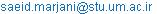(S. Marjani)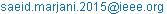(S. Marjani)

*Corresponding author

M. Dabbaghha, M. Dashtbayazi, S. Marjani, M. Sabaghi. Increased Accuracy in Image Segmentation Considering the Modular Method Based on Texture Characteristics and Super Pixel. Journal of Electrical and Electronic Engineering. Vol. 4, No. 3, 2016, pp. 63-67. doi: 10.11648/j.jeee.20160403.14

Received: May 13, 2016; Accepted: May 23, 2016; Published: June 4, 2016

Abstract: To solve the problem of segmenting an image into homogeneous regions with large area, this paper proposes an efficient algorithm that is based optimization base on modularity and super pixel method. Due to the fact that a very small areas of the image before segmentation, the proposed algorithm automatically merged with neighboring small areas and to make larger modules. When the modularity pictures after the merger to reach its maximum stop, leading to the production of segmentation algorithms are final. To keep repetitive patterns in a homogeneous area, a feature based on its histogram modularity with features like color and eventually identified two areas by creating a similarity matrix, it is suggested. So that the problem of segmentation and complexity of the problem to some extent eliminate in a way that due to the combined areas can be achieved for repetitive patterns. Simulation results show that the algorithm has good accuracy.

Keywords: Segmentation, Modularity, Super Pixel

Contents

1. Introduction

Due to the widespread use of digital cameras and smart phones than a few decades ago, these technologies are ubiquitous digital images taken as an urgent need to analyze the images, such as object recognition, profile and so as a very important step for a high level of image analysis and segmentation is required.. Before segmentation, spatial processing takes place which contains a set of pixels in homogeneous areas so significant that in a way that reduces the time complexity ,  image segmentation problems still remain for the following reasons , :

a) Segmentation of images in defining the problem and optimize the user and the affiliate program.

b) Image segmentation because of the large number of pixels in the images, especially for high-resolution pictures, very time consuming and it is the main obstacle is that the program must run in real-time. In addition, the grouping of several key factors that affect segmentation example: repetitive pattern, the relative size and proximity, and so on. All of these factors, and is intended to offer an efficient algorithm and, in addition, the division will also be presented in several different ways.

2. Related Work

Mean shift algorithm (MS): acts in a manner that image segmentation clustering with practice, and taking into account the probability density function in picture mode, each pixel location features common to attach a pixel color and location each of which converts into a vector and then mean shift method for applying spatial characteristics of each pixel is used. All the pixels, all those who are close to the point of convergence, two factors are evaluated by the bandwidth of its location, hs called and hr, the whole bandwidth, determine which piece is which. In addition to this minimum size segmentation is important to ensure the application in the process of segmentation is usually quick method however the problem is that it is very sensitive to parameters hr and hs. Since the density estimates for all pixels and features some small changes in the brightness of colors and textures or colors to different modes converge different rules, even if they are owned by the same vision

Watershed segmentation method (MCW):

This method is based on the slope of the topography of an image, in the image pixels that are drop shipped in a pond and intensity discharge the catchment area called it and it does not specify boundaries, but these methods generally are sensitive to noise and are not easily lead to segmentation with high accuracy , .

Module concept and detection in the field of network science has been highly regarded in the past decade and due to their widespread use in large-scale networks, we try to use this method in the segmentation of images in which millions of pixels to be included, be used.

In this section we examine these two concepts will be explained and then the latter method is based on the optimization of the measure in detail pay.

Modulo for the first time defined by M.E.J Newman  which is weighted network analysis. G is a weighted network and weighing adjacent matrix a measure Q for Eq. (1) are: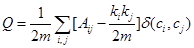(1)

Aij indicates that weight is between nodes I and nodes j and then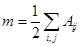, Represents the sum of network weight and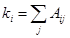is the degree of The node weight i, ci is the label of set in node I and so if In other words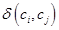is one or non-zero, nodes i and j in a community. In fact, module means the difference between real likely possibilities of connecting two nodes in a random connecting two nodes are set and estimate the probability. Other, Levin methods that measure on the basis of community detection, Increased modularity makes j community and an i community by equation (2) can be combined: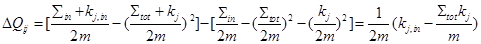(2)

And then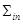are total weight of the edge is inside of i community and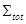are total weight of the edge node in community i.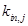is Total weight of the community i to community j. Other symbols in the above equation have the same definition. As explained below, the basic idea of Louvain method is to repeat the optimization process modules in the first phase and the second phase in the following categories:

1. Optimization modularity

The first phase of the network is composed of several community at the beginning of each community, a node and after a few repetitions of each community is a group of nodes) For each community i and connected to a node Ni ={j|Aij > 0}, and calculate the potential increase in modular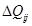, according to the above equation if we set our combined maximum modular resulting from the merger will be community i and j, now, if we repeat the process for complex networks, will not increase the modular.

2. Community Aggregation

For network reconstruction and consolidated community and those who shared the same label and tag them again heading The above process until the modular due to the merger of two, the other does not rise, continues. Our simulation method considers the following two aspects: 1-time complexity 2- maintaining order and precision that using software and community detection algorithms for large-scale network nodes, we look at the picture from the perspective of a network. For a network modules are a very important value . It is more accurate to say that the bigger modular, more precisely identifies the categories.

Considering the efficient calculation module in the set detection algorithm, which is a problem in image segmentation, and it must be said that the optimal segmentation is achieved when the modularity of the image is maximized. Although the modularity method for segmentation is used by researchers recently , . But other problems segmentation algorithms still remains above because of the ignorance of the intrinsic properties of images, unlike the algorithms that are based on our module the difference between community detection and image segmentation to identify. And the Super pixel start and one of the signs of low-level features for texture and visual characteristics of the object can be integrated and used regularly. And we similarity matrix code in addition, the similarity of pixel areas to avoid pre-segmentation has been made to the comparative method then Compared with other segmentation algorithms available, Our proposed algorithm can be automatically recognize some of the areas in the image and produce larger areas to maintain order and cohesion.

3. The Proposed Algorithm

The method that is used to network modularity method in this article for explorers because what we're here for updates divided areas is very similar things in telecommunication networks do for splitting nodes on specific areas to enhance network performance as shown in Figure 1.

Therefore, here too, we first color image, such as a random network node that here Super pixel they say, we divided. Super pixels of the array of pixels are almost the same features as the nodes of a network are us, and instead of that, let's examine the individual pixels come to consider a set of pixels. This will spend less time because in this way a small area and homogeneity of the pixels are initialized, they have no direct impact on the performance of segmentation. And also reduces the calculations which led to the rapid increase in performance. And also reduces the calculations which led to the rapid increase in performance. Thus, a pre-processing step for us, at first point we should consider super pixel’s, then this super pixel’s, we can produces with K Clustering algorithm (we can choose K upper than 200).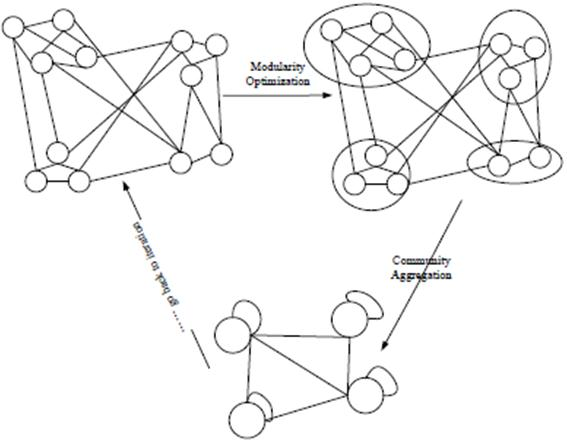Fig. 1. Structure of the network and the selection of modules.

Our approach in the implementation of algorithms that create a super pixel that is publicly available and have been used . Then, using an iterative algorithm to the cloud we are trying to classify super pixels in separate areas and each time we update this division so as to reach a position where other areas of updates, no substantial change That at this stage we can say that image segmentation is the best way. It should be noted that this classification is based on a similarity matrix of rows and columns that it features areas to form them. At each stage, segment of image features are extracted and then update the similarity matrix algorithm based on the similarity of their members in the areas of newer markets. The calculation method of a similarity matrix is as follows.

In conventional luvin’s methods for community detection of a similarity matrix is updated on a constant basis for the new similarity matrix used, by weight of nodes in two different sets during each iteration, done. We are proposing to construct adaptive similarity matrix is as follows: The matrix array of colors and textures are two factors which has to transfer the color distribution of the Gaussian equation (3) we use: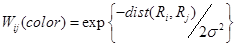(3)

That dist (Ri, Rj), The distance between the distribution areas Ri and Rj, In all computation use of average distance and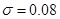histogram State (HOS) per area by a 256-dimensional vector is shown meaning that Ri and Rj areas HOS feature vector is as follows: hi, hj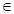R256, We cosine similarity measure for texture features are: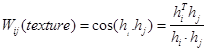(4)

Now for computing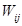, various methods of network segmentation for different methods used to obtain them. But in this article from conventional hybrid model, we used that relationship in the form of equation (5) is: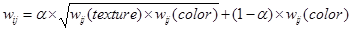(5)

If the above equation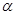is equal to zero, such that the image is clear borders, Such as images of parallel lines, However, if the amount ofis large, In the picture tree with grass merge and form a piece that, it makes the border between grass and trees are not specified , .

Now that is what’s the being discussed on the features of the image feature extraction, so this method works best to show, nature can be a lot of work done on this topic because today a lot of features for image definition and There are also many ways and But the way in which this paper is based on the HOS  image Instead of making gradient histogram is that we create a histogram State (HOS) will follow three steps for each area.

1. Calculate the amount of tilt and 360 degree orientation information for each pixel in an image

2. In each pixel location of a window 5 x 5 is used for sliding and a histogram inclined by 360° orientation is divided into 8 modules Each pixel is then provided to a seven-dimensional vector orientation and With each dimension indicate whether the orientation of the sliding window is already there, That is the context information for each pixel belonging to one of the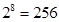modes.

3. We have a histogram for each region will build 256 next of the state vector and each piece specifies the number of such cases and then we normalized by the number of pixels in that section.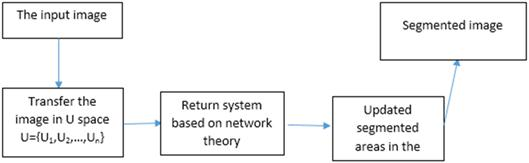Fig. 2. The flowchart of the proposed algorithm.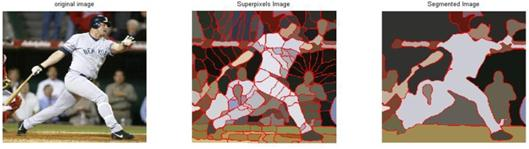Fig. 3. Images segmentation with the proposed algorithm.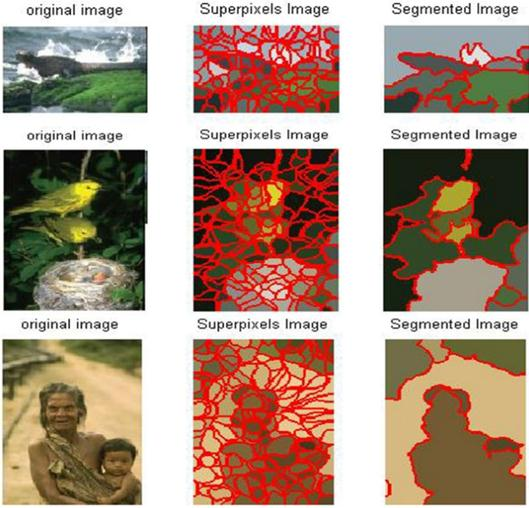Fig. 4. Images segmentation with the proposed algorithm.

The proposed algorithm has been tested on various data and reference use these pictures: The official website of UC Berkeley's existing database on the topic segmentation (BSDS500) and other database called Semantic Segmentation Data Set (SSDS) The proposed algorithm in Matlab and by laptop with intel core i7 processor and ATI graphics card and 8GB RAM is done.

4. Quantitative Evaluation

To evaluate the efficiency of the proposed method is very useful two criteria examine the quality of segmentation methods described below can be used to arrange:

a) The first criterion is Probabilistic Rand Index (PRI) which represents the probability that a sample pairs in two segmentation have the same labels that this is a number between zero and one that is much larger indicating similarity between the two segmentation .

b) The second measure is the Variation of Information (VOI) how can we know the information using segmentation, the segmentation other information we have that kind of lack of independence shows that no matter how less is better.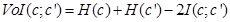(6)

Where H (C) and H (C ') are represent the entropy segmentation of C' and C,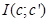It is an expression of mutual information between the two segmentation. The numerical value between zero and infinity these criteria can be changed. Also, because the above two criteria proposed algorithm was one of the better and another be worse.

So, we decided to compare more than two important benchmark in the field of data mining include the use of precision and recall do  Let ctest represents segmentation been tested and Cgt represents real segmentation (ground-truth) The accuracy of the segmentation is determined by the following formula and indicates that not true scope of pixels found to belong or not to have gone wrong.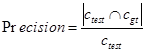(7)

In this case, |C| Part C shows the number of pixels in the area. The next criterion is to recall that the pixels that have been identified within the actual show.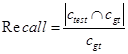(8)

To summarize the results of these two criteria can be use eq (9) Fisher-alpha, which in total represent the performance of the system. Here parameter alpha was 0.5 equal the impact of these two factors ultimately be the same.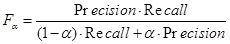(9)

The results indicate that the system has improved in terms of accuracy.

Table 1. Quantitive comparison of different algorithms on BSDS.

 Algorithms PRI (larger better) VOI (smaller better) Our’s 0.732 2.151 MCW  0.753 2.203

Table 2. Quantitive comparison of different algorithms on SSDS.

 Algorithms precision recall F0.5-measure Our’s 0.292 0.322 0.306 MCW 0.228 0.342 0.274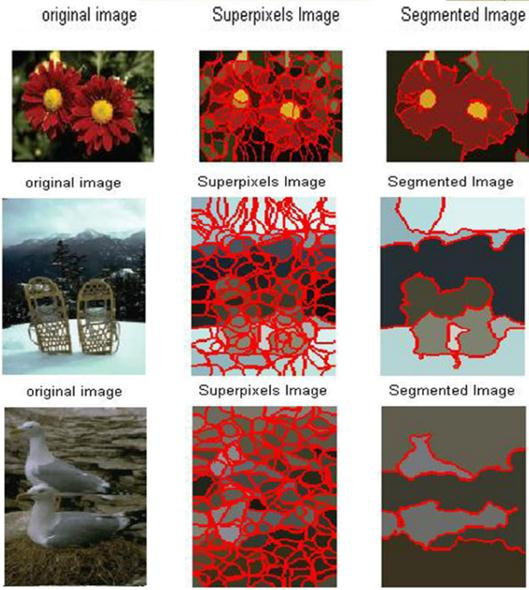Fig. 5. Images segmentation with the proposed algorithm.

5. Conclusion

In this paper, an efficient segmentation algorithm was proposed to measure and optimize the intrinsic properties of the image and the image segmentation using super pixels focuses and automatically according to the characteristics of the color histogram of State (HOS) in the process to be handled And optimized matrix similarity between different regions and adjacent areas to gather regularly measure And so long until the conformity with adjacent collects, So that the module does not increase. Which leads to good quality segmented and at the end with a segmentation method, two criteria were compared and VOI in a parameter, the algorithm was improved and we will be one of two measures improved the VOI = 2.151 and F0.5-measure = 0.306, which is indicative of increased accuracy in segmentation.

References

1. B. Bhanu and J. Peng, "Adaptive integrated image segmentation and object recognition", IEEE Transactions on Systems, Man, and Cybernetics, Part C: Applications and Reviews, vol. 30, no. 4, pp. 427–441, 2000.
2. H. Zhu, J. Zheng, J. Cai, and N. M. Thalmann, "Object-level image segmentation using low level cues", IEEE Transactions on Image Processing, 2013.
3. M. Wertheimer, "Laws of organization in perceptual forms," A Source Book of Gestalt Psychology, pp. 71–88, 1938.
4. D. D. Hoffman and M. Singh, "Salience of visual parts," Cognition, vol. 63, no. 1, pp. 29–78, 1997.
5. L. Vincent and P. Soille, "Watersheds in digital spaces: an efficient algorithm based on immersion simulations", IEEE transactions on Pattern Analysis and Machine Intelligence, vol. 13, no. 6, pp. 583–598, 1991.
6. V. Osma-Ruiz, J. I. Godino-Llorente, N. S´aenz-Lech´on, and P. G´omez- Vilda, "An improved watershed algorithm based on efficient computation of shortest paths", Pattern Recognition, vol. 40, no. 3, pp. 1078–1090, 2007.
7. D. Martin, C. Fowlkes, D. Tal, and J. Malik, "A database of human segmented natural images and its application to evaluating segmentation algorithms and measuring ecological statistics", The Proceedings of the 8th IEEE International Conference on Computer Vision, ICCV, vol. 2, pp. 416–423, July 2001.
8. M. Newman, "Analysis of weighted networks", Physical Review E, vol. 70, no. 5, p. 056131, 2004.
9. A. Browet, P.-A. Absil, and P. Van Dooren, "Community detection for hierarchical image segmentation", Combinatorial Image Analysis . Springer, pp. 358–371, 2011.
10. W. Li, "Modularity segmentation", Neural Information Processing. Springer, pp. 100–107, 2013 .
11. G. Mori, "Guiding model search using segmentation," The Proceedings of the 10th IEEE International Conference on Computer Vision, ICCV, vol. 2, pp. 1417–1423, 2005.
12. D. Dowson and B. Landau, "The frechet distance between multivariate, normal distribution", Jornal of multivariate Analysis, vol 12, no 3, pp. 450 455, 1982.
13. J. Puzicha, J. Buhmann, Y. Rubner, and C. Tomasi, "Empirical evaluation of dissimilarity measures for color and texture", The Proceedings of the 7th IEEE International Conference on Computer Vision, ICCV, Kerkyra, Greece, pp. 1165–1172, 1992.
14. N. Dalal and B. Triggs, "Histograms of oriented gradients for human detection" IEEE Conference on Computer Vision and Pattern Recognition, CVPR , vol. 1, pp. 886–893, 2005.
15. D. R. Martin, c. c. Fowlkes, and J. Malik, "Learning to detect natural image boundaries, color, andtexture cues", IEEE Transaction on pattern Analysis and machine intelligence, vol, 26, no. 5, pp. 530–549, 2004.
16. A. Yang, J. Wright, Y. Ma, and S. Sastry, "Unsupervised segmentation of natural image via lossy data compression", Computer version and image understanding, vol 110, no 2, pp. 212–225, 2008.
17. C. Couprie, L. Grady. L. Najman, and H. Talbot, "Power watershed: A unifying graph-based optimization framework," IEEE Transaction on Pattern analysis and Machine Intelligence, vol. 33, no. 7, pp. 1384–1399. 2011.

 Contents 1. 2. 3. 4. 5.
Article ToolsAbstractPDF(938K)